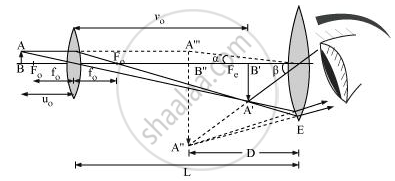Department of Pre-University Education, KarnatakaPUC Karnataka Science Class 12
Advertisement Remove all ads

# Draw a Ray Diagram to Show the Working of a Compound Microscope. Deduce an Expression for the Total Magnification When the Final Image is Formed at the Near Point.In a Compound Microscope, an Obje - Physics

Advertisement Remove all ads
Advertisement Remove all ads
Advertisement Remove all ads

Draw a ray diagram to show the working of a compound microscope. Deduce an expression for the total magnification when the final image is formed at the near point.

In a compound microscope, an object is placed at a distance of 1.5 cm from the objective of focal length 1.25 cm. If the eye piece has a focal length of 5 cm and the final image is formed at the near point, estimate the magnifying power of the microscope.

Advertisement Remove all ads

#### Solution

Ray diagram for a compound microscopeTotal angular magnification, m = beta/alpha

β → Angle subtended by the image

α → Angle subtended by the object

Since α and β are small,

tan alpha ≈ alpha and tan beta ≈ beta

m= (tanbeta)/(tanalpha)

tan alpha = (AB)/D

And

tan beta = (A''B'')/D

m = (tanbeta)/(tanalpha) = (A''B'')/D xx D/(AB) = (A''B'')/(AB)

On multiplying the numerator and the denominator with A′B′, we obtain

m = (A''B'' xx A'B')/(A'B' xx AB)

Now, magnification produced by objective, m_0 = (A'B')/(AB)

Magnification produced by eyepiece, m_e = (A''B'')/(AB)

Therefore,

Total magnification, (m) = m0 me

m_0 = ( V_0) / (u_0) =(\text { Image distance for image produced by objective lens})/(\text { Object distance for the objective lens})

m_e = (1+D/(f_e))

f→ Focal length of eyepiece

m = m_0m_e

= V_0/u_0(1+D/f_e)

V_0 ≈ L(Separation between the lenses)

u_0 ≈ -f_0

therefore m = (-L)/(f_0) (1 +D/f_e)

u_0 = -1.5 cm

f_0 = +1.5cm

1/f_0   = 1/v_0 - 1/u_0

1/1.25 =1/v_0 + 1/1.5

1/v_0=1/1.25 - 1/1.5

= 100/125 - 10/15

= (1500 -1250)/1875

1/v_0 = 250/1875

v_0 = + 7.5 cm

f_e = + 5cm

m =v_0/u_0 (1+D/f_e)

= 7.5 / - 1.5 (1+25/5)

= - 7.5/1.5 xx 6

m =-30

Concept: Optical Instruments - The Microscope
Is there an error in this question or solution?

#### Video TutorialsVIEW ALL 

Advertisement Remove all ads
Share
Notifications

View all notifications

Forgot password?